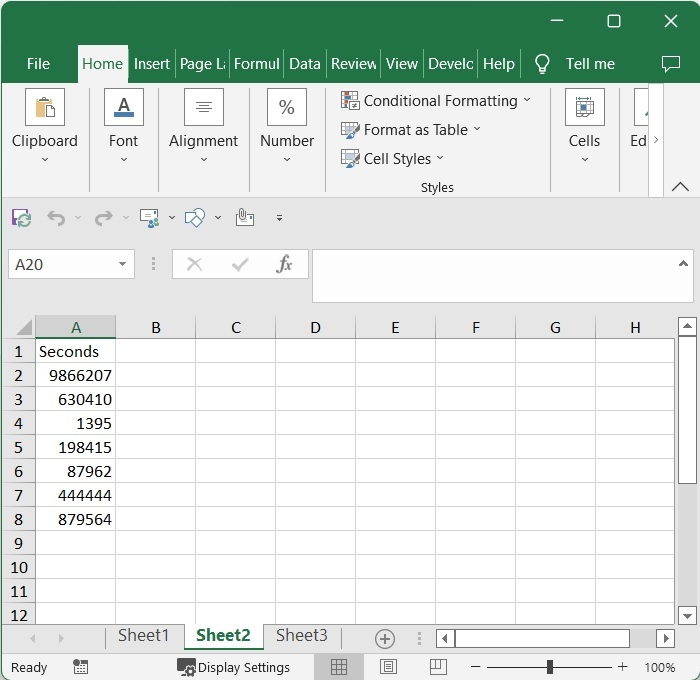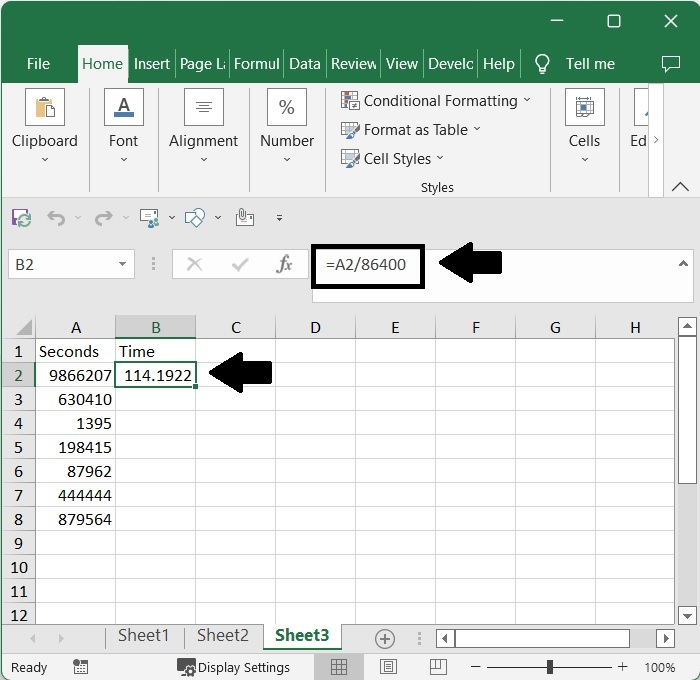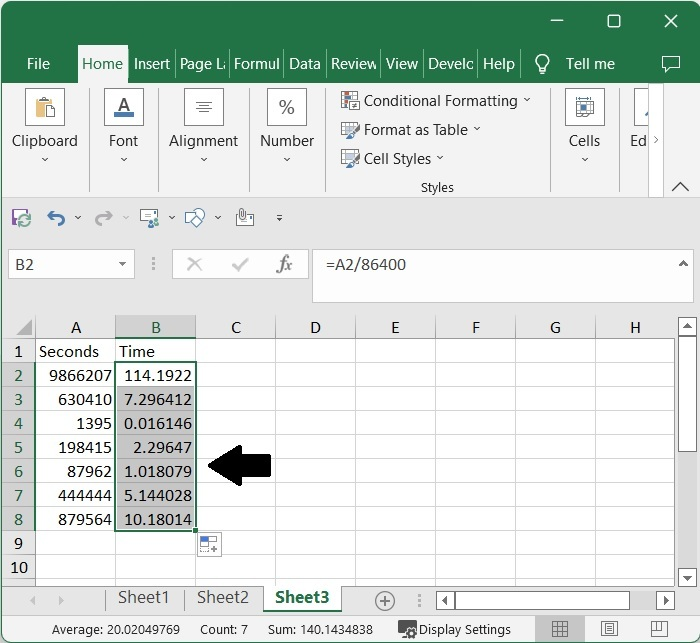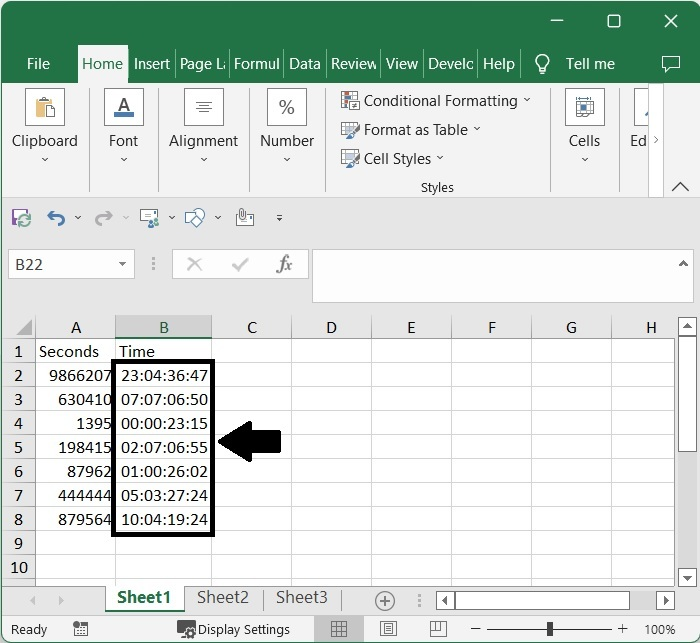# How to Convert Seconds to Time (hh mm ss) or Vice Versa in Excel?

The data in the sheet can be very confusing if it is presented in seconds, and the value will be so large that you won't be able to perform any operations on it. A second typically corresponds to 1/3600 of an hour. We can use this method to determine the duration in seconds. You can learn how to convert seconds to time in Excel from the information provided in this tutorial. In this tutorial, we'll try to use the formulas to convert seconds to duration. Although a direct formula is not yet available, we can create one by simply comprehending our data.

## Converting Seconds to Time (hh mm ss)

In this case, we'll use the formula to get one result and then use the autofill handle to get all of the other results. Let’s look at a simple procedure for converting seconds to time in Excel.

### Step 1

Let us consider an Excel sheet where the data is a list of seconds, similar to the below image.Now we need to convert seconds into days. To do so, click on an empty cell, in our case cell B2, and enter the formula as =A2/86400 and click Enter to get our first value, which looks similar to the below image.### Step 2

Then drag down from the first result using the auto-fill handle to get all the values as shown in the below image.As we can see, the values are shown in decimals. We need to format them to get our final result.

### Step 3

Now select the generated values and right-click, then select format cells to open a pop-up window. In the pop-up, click on "Custom" and enter the type as dd:hh:mm:ss, then click "OK" to complete our process.## Conclusion

In this tutorial, we used a simple example to demonstrate how you can convert seconds to time in Excel.